ISEE Middle Level Math : Quadrilaterals

Example Questions

Example Question #25 : How To Find The Area Of A Rectangle

Jeff decided to build a play area for his guinea pigs. The play area would be an enclosure 6 feet long, 2 feet wide, and 2 feet tall. In cubic feet, how big is the play area?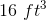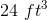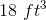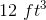Explanation:

The cubic feet of an area is found by multiping the length times the width times the height. Given that the length is six feet, the width is two feet, and that the height is two feet, the total cubic area would be found using this equation: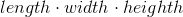Here is the equation with the appropriate numbers plugged in: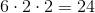Therefore, 24 cubic feet is the correct answer.

Example Question #6 : Geometry

Jessica's blanket is 12 square feet. Lisa has a blanket that is half the size of Jessica's blanket. Which of the following are possible dimensions of Lisa's blanket?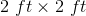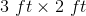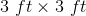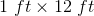Explanation:

The area of a rectangle if found by multiplying the length times the width. Here, we know that Lisa's blanket is half the area of Jessica's blanket. Since Jessica's blanket is 12 square feet, that means that Lisa's blanket must be 6 square feet.

The only length and width values that give us 6 square feet when multiplied by one another are 3 feet by 2 feet. This is therefore the correct answer.

Example Question #11 : Rectangles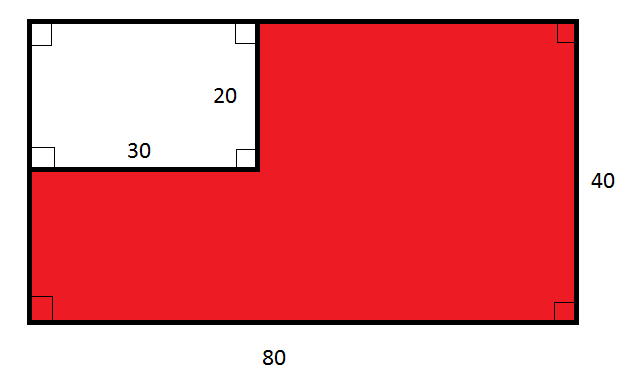Note: Figure NOT drawn to scale.

What percent of the above figure is red?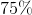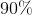The correct answer is not given among the other choices.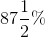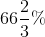The correct answer is not given among the other choices.

Explanation:

The large rectangle has length 80 and width 40, and, consequently, area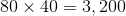.

The white region is a rectangle with length 30 and width 20, and, consequently, area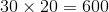.

The red region, therefore, has area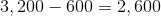The red region is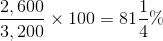of the large rectangle.

This is not one of the choices.

Example Question #27 : How To Find The Area Of A RectangleNote: Figure NOT drawn to scale.

Refer to the above diagram. Give the ratio of the area of the red region to that of the white region.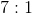The correct answer is not given among the other choices.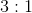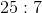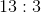Explanation:

The large rectangle has length 80 and width 40, and, consequently, area.

The white region is a rectangle with length 30 and width 20, and, consequently, area.

The red region, therefore, has areaThe ratio of the area of the red region to that of the white region is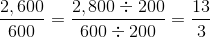That is, 13 to 3.

Example Question #28 : How To Find The Area Of A Rectangle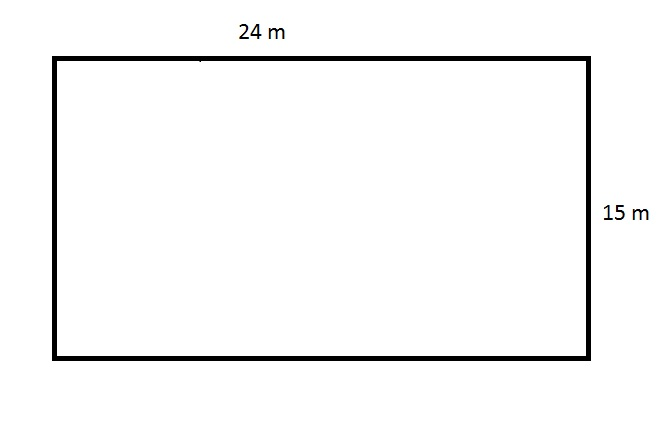The above figure depicts the rectangular swimming pool at an apartment. The apartment manager needs to purchase a tarp that will cover this pool completely, but the store will only sell the material in multiples of one hundred square meters. How many square meters will the manager need to buy?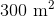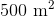Insufficient information is given to answer the question.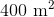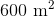Explanation:

The tarp needed to cover this pool must be, at minimum, the product of its length and width, or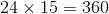square meters.

The manager will need to buy a number of square yards of tarp equal to the next highest multiple of one hundred, which is 400 square meters.

Example Question #31 : How To Find The Area Of A Rectangle

The four angles of a square are labeled A, B, C, and D. What is the sum of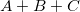?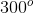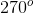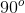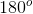Explanation:

In a square, each angle is 90 degrees.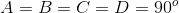We can plug in 90 for each variable and find the sum.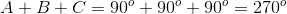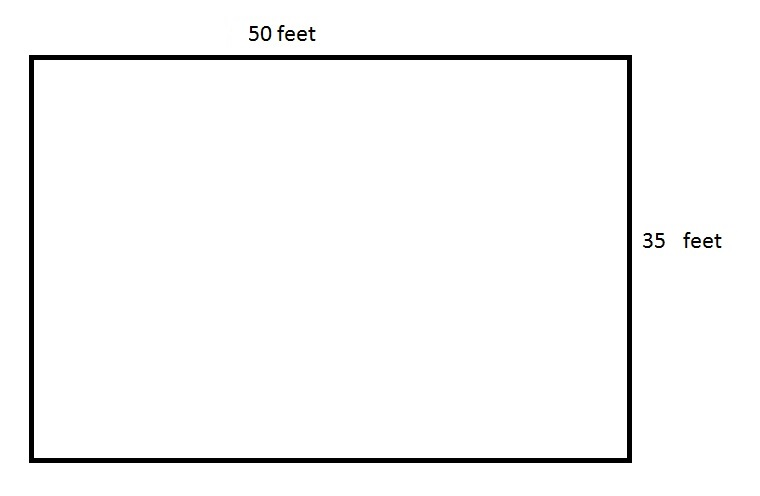The above depicts a rectangular swimming pool for an apartment. The pool is six feet deep everywhere.

An apartment manager wants to paint the four sides and the bottom of the swimming pool. How many square feet will he need to paint?

The correct answer is not given among the other responses.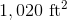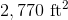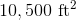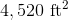Explanation:

The bottom of the swimming pool has area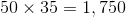square feet.

There are two sides whose area is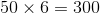square feet,

and two sides whose area is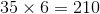square feet.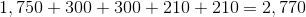square feet.

If the angles of a quadrilateral are equal to,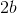,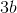, and, what is the value of?Explanation:

Given that there are 360 degrees in a quadrilateral,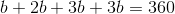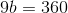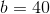What is the value of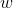if the angles of a quadrilateral are equal todegrees,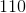degrees,degrees, and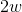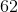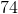Explanation:

Given that there are 360 degrees in a quadrilateral,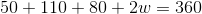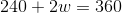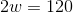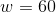If the length of a rectangle is 7.5 feet and the width is 2 feet, what is the value ofif the area is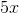?Given that the area is also equal to, the value ofwill be 3, given that 3 times 5 is 15.